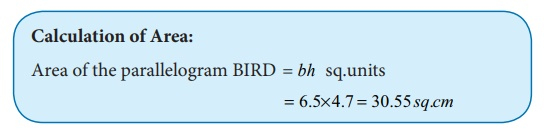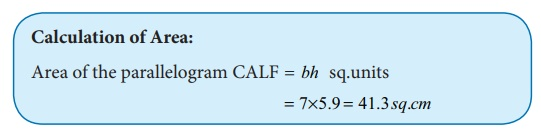Home | | Maths 8th Std | Construction of a Parallelogram

# Construction of a Parallelogram

Let us construct a parallelogram with the given measurements 1. Two adjacent sides and one angle. 2. Two adjacent sides and one diagonal. 3. Two diagonals and one included angle. 4. One side, one diagonal and one angle.

Construction of a Parallelogram

Let us construct a parallelogram with the given measurements

1. Two adjacent sides and one angle.

2. Two adjacent sides and one diagonal.

3. Two diagonals and one included angle.

4. One side, one diagonal and one angle.1. Constructing a parallelogram when its two adjacent sides and one angle are given

Example 5.30

Construct a parallelogram BIRD with BI=6.5cm, IR=5cm and BIR = 70º . Also find its area.

Solution:

Given:

BI=6.5cm, IR=5cm and BIR = 70ºSteps:

1. Draw a line segment BI=6.5cm.

2. Make an angle BIX = 70º at I on ̅B̅I̅

3. With I as centre, draw an arc of radius 5cm cutting IX at R.

4. With B and R as centres, draw arcs of radii 5cm and 6.5cm respectively. Let them cut at D.

5. Join BD and RD.

6. BIRD is the required parallelogram.

Calculation of Area:

Area of the parallelogram BIRD = bh sq.units

= 6.5× 4.7 = 30.55 sq.cm2. Constructing a parallelogram when its two adjacent sides and one diagonal are given

Example 5.31

Construct a parallelogram CALF with CA=7cm, CF=6cm and AF=10cm. Also find its area.

Solution:

Given:

CA=7cm, CF=6cm and AF=10cmSteps:

1. Draw a line segment CA=7cm.

2. With C and A as centres, draw arcs of radii 6cm and 10cm respectively. Let them cut at F.

3. Join CF and AF.

4. With A and F as centres, draw arcs of radii 6cm and 7cm respectively. Let them cut at L.

5. Join AL and FL.

6. CALF is the required parallelogram.

Calculation of Area:

Area of the parallelogram CALF = bh sq.units

= 7×5.9 = 41.3 sq.cm3. Constructing a parallelogram when its two diagonals and one included angle are given

Example 5.32

Construct a parallelogram ∆UCK with DC=8cm, UK=6cm and DOU =110º . Also find its area.

Solution:

Given:

DC=8cm, UK=6cm and DOU =110ºSteps:

1. Draw a line segment DC=8cm.

2. Mark O the midpoint of D̅C̅

3. Draw a line X̅Y̅  through O which makes DOY = 110º .

4. With O as centre and 3cm as radius draw two arcs on  ̅X̅Y̅  on either sides of D̅C̅ . Let the arcs cut O̅X̅at K and O̅Y̅at U

5. Join D̅U̅, U̅C̅, C̅K̅and K̅D̅.

6. DUCK is the required parallelogram.

Calculation of Area:

Area of the parallelogram DUCK = bh sq.units

= 5.8 × 3.9 = 22.62 sq.cm4. Constructing a parallelogram when its one side, one diagonal and one angle are given

Example 5.33

Construct a parallelogram BEAR with BE=7cm, BA=7.5cm and BEA = 80º . Also find its area.

Solution:

Given:

BE=7cm, BA=7.5cm and BEA = 80°Steps:

1. Draw a line segment BE=7cm.

2. Make an angle BEX = 80º at E on B̅E̅.

3. With B as centre, draw an arc of radius 7.5cm cutting EX at A and Join BA.

4. With B as centre, draw an arc of radius equal to the length of A̅E̅.

5. With A as centre, draw an arc of radius 7cm. Let both arcs cut at R.

6. Join BR and AR.

7. BEAR is the required parallelogram.

Calculation of Area:

Area of the parallelogram BEAR = bh sq.units

= 7×4.1 = 28.7 sq.cmTags : Questions with Answers, Solution | Geometry | Chapter 5 | 8th Maths , 8th Maths : Chapter 5 : Geometry
Study Material, Lecturing Notes, Assignment, Reference, Wiki description explanation, brief detail
8th Maths : Chapter 5 : Geometry : Construction of a Parallelogram | Questions with Answers, Solution | Geometry | Chapter 5 | 8th Maths